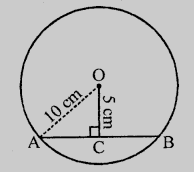# Find the length of a chord which is at a distance of $5\ cm$ from the centre of a circle of radius $10\ cm$.

Given:

A chord is at a distance of $5\ cm$ from the centre of a circle of radius $10\ cm$.

To do:

We have to find the length of the chord.

Solution:Let $AB$ be a chord of a circle with radius $10\ cm$.

$OC \perp AB$

This implies,

$OA = 10\ cm$

$OC = 5\ cm$

$OC$ divides $AB$ into two equal parts.

$AC = CB$

In right angled triangle $OAC$,

$OA^2 = OC^2 + AC^2$                 (Pythagoras Theorem)

$(10)^2 = (5)^2 + AC^2$

$100 = 25 + AC^2$

$AC^2 = 100 - 25 = 75$

$\Rightarrow AC = \sqrt{75}$

$= \sqrt{25\times3}$

$= 5 \times 1.732$

$AB = 2 \times AC = 2 \times 5 \times 1.732$

$= 10 \times 1.732$

$= 17.32\ cm$

The length of the chord is $17.32\ cm$.

Updated on: 10-Oct-2022

88 Views